Equation Checks
for Multiple Choice Questions

The graphing calculator allows for several methods of checking or comparing multiple choice answers.  Let's look at the checking of algebraic problems by forming equations.

Process:

 Choose your "favorite" positive one-digit (for ease) integer value and store the value in x (do not pick 0 or 1).   To store a 7:   7 STO (key) x Hit ENTER.  Enter the algebraic expression from the problem on the home screen using x as your variable. Set this expression "=" to the each of the multiple choice answers. The "=" sign is under 2nd TEST (MATH) #1 =. Hit ENTER. If a 0 appears, this is NOT the correct answer. If a 1 appears, this IS the correct answer.
 The "recall entry" feature of the calculator can be used to speed up data entry.  (2nd ENTER)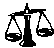1. The expression (x - 6)2 is equivalent to      (1) x2 - 36      (2) x2 + 36      (3) x2 - 12x + 36      (4) x2 + 12x + 36The "favorite" number used here was 7.  When you hit ENTER and a "1" appears, you have found the answer (the equation is "true").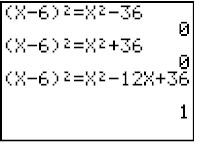2.  Which of the following statements is true?      (1)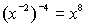(2)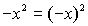(3)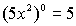(4)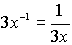When in doubt, use parentheses when entering information.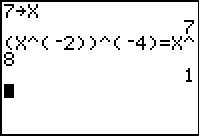3.  When 12x3 + 4x is divided by 4x, the answer is      (1) 3x2 + 1      (2) 3x + 1      (3) 3x2 + x      (4) 3x2 Parentheses are needed for both the numerator and the denominator in the division of this problem.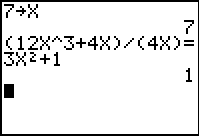Finding Your Way Around TABLE of  CONTENTS